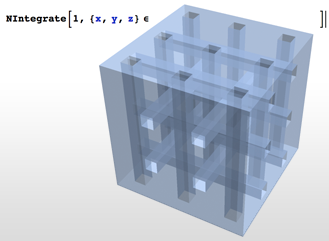# Symbolic Geometry

Version 10 adds broad support for geometric computation, with the key element being that of geometric region. Geometric regions can be created by using special regions such as circle, using formulas, using meshes containing collections of simple regions, or combining other regions through Boolean combinations and transformations. Several standard properties can be directly computed for every region, such as membership tests, measure (e.g. length, area, volume), centroid, nearest points, etc. Regions can also be used as specifications for many high-level solvers, including optimization, algebraic equation solving, integration, and partial differential equation solving.• Introduce geometric regions as first-class citizens in the Wolfram Language.
• Full support for a large number of basic and common special regions. »
• Full support for formula regions either implicit or explicit (parametric). »
• Full support for mesh-based regions in 1D, 2D, and 3D. »
• Full support for derived regions such as Boolean combinations and transformations. »
• Support for computing measure (length, area, volume, etc.) for any region, (approximately, exactly, and with parameters).
• Support for computing centroids for any region (approximately, exactly, and with parameters).
• Support for computing nearest points, distance, and signed distance from a point to any region (approximately, exactly, and with parameters).
• Support for testing membership and conditions for membership for any region.
• Support for integration over any region (approximately, exactly, and with parameters).
• Support for solving partial differential equations and systems of partial differential equations over regions using finite elements.
• Support for optimization using region constraints (approximately and exactly).
• Support for solving equations (approximately and exactly), inequalities, and quantifiers (exactly and with parameters) using region constraints.
• Support for regions of any geometric dimension less than the embedding dimension, e.g. points, curves, and surfaces in 3D or regions including mixed-dimension elements.
• Support for non-manifold regions. In fact, manifolds are never a requirement.
• Support for special and formula regions in any dimension.
• Automatic region construction from point sets, including Delaunay triangulated meshes, Voronoi tessellated meshes, and convex hulls.
• Automatic discretization of 2D and 3D graphics to mesh-based regions.
• Automatic discretization of any 1D, 2D, and 3D embedded region to mesh-based regions.
• Automatic triangulation of mesh-based regions in 1D, 2D, and 3D.

## Mathematica

Questions? Comments? Contact a Wolfram expert »